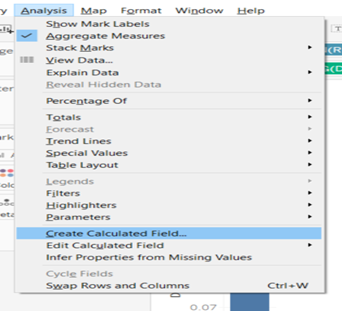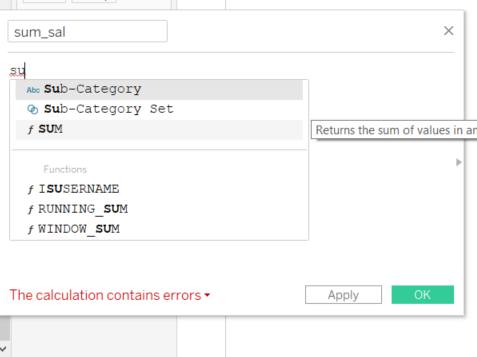top of pageSearch

# Aggregate Functions in TableauAggregate Functions are a type of function where values of multiple rows are grouped together as input and output a single value.

Below is the list of aggregate functions available in Tableau:

SUM: returns the total of all values in a measure. Null values are ignored

AVG: returns the average of all numbers in a measure. Null values are ignored.

MEDIAN: returns the median of the values.

MIN: returns the minimum of values

MAX: returns the maximum of values

PERCENTILE: returns the “kth percentile” for a set of data. A percentile is a value below which a given percentage of values in a data set fall.

VAR: This is a statistical function. VAR will return the statistical variance of all the values based on the sample population.

VARP: It is similar to VAR, but it will return the statistical variance of all the values based on the entire population.

STDEV: standard deviation is the statistical function; it will return the standard deviation for all the values on the sample population.

STDEVP: It is similar to STDEV, this will return the standard deviation for all the values on the entire population.

COUNT: returns the number of items in a group. Null values are not counted.

COUNTD: returns the distinct items in a group. Each unique item is counted only once.

Aggregate functions can be used both on dimensions and measures.

Aggregating Measures:

When we add Measures to the view, an aggregation is applied to those measures by default. Average, Sum, and Median are the common aggregate functions. As shown in Fig 1

If we want to change the default aggregation just right-click on that field and select the default properties.

Let me explain this with an example:

Drag Region to the Columns and Sales to the Rows, we can observe Sales become SUM(Sales). As shown in Fig 1.

We can change the default aggregation by just right-clicking on Sum(Sales) and selecting any of the options. I am selecting Average here, as shown in Fig 2.Fig 1 Fig 2

To change the default aggregation right-click on a “data field” in Measure from the pane. For example click on the Discount data field and select “Default Properties” then select “Aggregation” and then select the aggregation options. As shown in Fig 3Fig 3

Disaggregate the data:

When we add a measure to the view, aggregation is applied automatically. This default is controlled by the “Aggregate Measures” settings in the “Analysis” menu. As shown in Fig 4.

If we want to see the detailed level of the data, that means a separate mark for every value in every row of the data set, we can go to the “Analysis menu” and uncheck the” Aggregate Measures”. We can see the changes as shown in Fig 5.Fig 4 Fig 5

Usage of Aggregate Functions in Calculated Field.

Go to the Analysis tab, click on Create calculated Field option (Fig 6), a new window will be opened(Fig 7). Rename the default calculation name.Fig 6 Fig 7

Let me explain this with the “Sum” aggregate function

We will calculate the sum of sales for superstore data.

Let’s create a calculated field “sum_sal”, as shown in fig 8 when we type Tableau aggregate calculation window will show us the suggestions for the functions.

Click Apply and Ok.

Fig 8 Fig 9A new calculated field “sum” is created. The calculated field will be shown as a “.#”

As shown in Fig 10.Fig 10

Similarly, all the other Aggregate functions can be used within calculated fields for better analysis and to create in-depth visualizations.